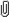June 09, 2023, 04:46:19 pm

###AuthorTopic: Please help!!  (Read 2253 times) Tweet Share

0 Members and 1 Guest are viewing this topic.

#### s0m30n312345

•• Posts: 8
• Respect: 0« on: February 27, 2022, 02:46:20 pm »
0

1. A family of parabolas satisfies the rule y = ax^2 + 2x + a.
a) Express ax^2 +2x+a in the format a(x+b)^2 +c for real numbers b and c.
b) For which values of a is ax^2+2x+a a perfect square?
c) For which values of a does the graph of y=ax^2+2x+a have two x-axis intercepts?

2. Write down four quadratic rules that have graphs similar to those in the diagram.

3. Find quadratic expressions which could represent the two curves in this diagram, given that the coefficient of x is 1 in each case. The labelled points are A(2, 3), B(2, 1), C(0, −5) 2 and D(0, 2).

4. The rate of rainfall during a storm t hours after it began was 3 mm per hour when t = 5, 6 mm per hour when t = 9 and 5 mm per hour when t = 13. Assuming that a quadratic model applies, find an expression for the rate of rainfall, r mm per hour, in terms of t.

#### 1729

• MOTM: July 20
• Trendsetter
•• Posts: 185
• Respect: +158« Reply #1 on: February 27, 2022, 07:22:51 pm »
+1

1. A family of parabolas satisfies the rule y = ax^2 + 2x + a.
a) Express ax^2 +2x+a in the format a(x+b)^2 +c for real numbers b and c.
b) For which values of a is ax^2+2x+a a perfect square?
c) For which values of a does the graph of y=ax^2+2x+a have two x-axis intercepts?

2. Write down four quadratic rules that have graphs similar to those in the diagram.

3. Find quadratic expressions which could represent the two curves in this diagram, given that the coefficient of x is 1 in each case. The labelled points are A(2, 3), B(2, 1), C(0, −5) 2 and D(0, 2).

4. The rate of rainfall during a storm t hours after it began was 3 mm per hour when t = 5, 6 mm per hour when t = 9 and 5 mm per hour when t = 13. Assuming that a quadratic model applies, find an expression for the rate of rainfall, r mm per hour, in terms of t.

Hey - I have written solutions below for you but in the future it would be a lot easier for other people to help if you show your attempt or progress at the question - (totally fine if you are not too sure where to start with the question but try and tell us what you don't understand about it)

Question 1
$\mathbf{a.}\quad$ Note that you may only complete the square with monic quadratics so I have factorised the expression as such
$y=ax^{2}+2x+a$
$y=a\left(x^{2}+\frac{2}{a}x\right)+a$ (factor $a$ out of the function so we can complete the square)
$y=a\left(x^{2}+\frac{2}{a}x+\left(\frac{1}{a}\right)^{2}\right)+a-a\left(\frac{1}{a}\right)^{2}$ (C-Square for the expression inside the brackets and take out value inside bracket to make equivalent)
$y=a\left(x+\frac{1}{a}\right)^2+\frac{a^2-1}{a}$ (simplification of above)

$\mathbf{b.}\quad$ A function is considered to be perfect square if it can be written as $\left(x+c\right)^2$.
If the above can be written as $\left(\sqrt{a}\left(x+\frac{1}{a}\right)\right)^2+\frac{a^2-1}{a}$ what values of $a$ will make it a perfect square?
$\frac{a^2-1}{a}=0$. Alternatively, you could solve for when $\Delta =0$ as perfect square implies that the functions vertex lies on the $x$ axis.

$\mathbf{c.}\quad$ For this question you could either solve $\Delta >0$ or recognize the effects of adjusting $a$ for the graph in vertex form. (consider what vertical translation needs to happen in order for there to be 2 solutions for when the parabola is open upwards and downwards)

Quesiton 2
What have you tried here? Some hints for this question - try substituting corresponding $x$ and $y$ values for the following quadratic forms of parabolas (you need to consider which form is most appropriate for each graph with the coordinates given)
- $y=a\left(x-h\right)^{2}+k$
- $y=a\left(x-e\right)\left(x-f\right)$
- $y=ax^{2}+bx+c$

Quesiton 3
The above advice applies here - substitute coordinates for $y=ax^{2}+x+c$
If you do not know what I mean by "substitue coordinates" - if you were to substitute $A\left(2,3\right)$ in the above equation it would look something like $3=a\left(2\right)^{2}+\left(2\right)+c$

Question 4
Let $r$ denote the rate of rainfall in mm per hour and $t$ denote time in hours.
Given a quadratic model applies, the graph/function will be in the form of $r=at^{2}+bt+c$
Here, you will substitute the following values to get $a$, $b$ and $c$
- $r=3,\:t=5\Rightarrow 3=a\left(5\right)^2+b\left(5\right)+c$
- $r=6,\:t=9\Rightarrow 6=a\left(9\right)^2+b\left(9\right)+c$
- $r=5,\:t=13\Rightarrow 6=a\left(13\right)^2+b\left(13\right)+c$
Now solve the system of equations.

I hope this helps, and don't hesitate to ask for help if it doesn't#### s0m30n312345

•• Posts: 8
• Respect: 0« Reply #2 on: February 27, 2022, 10:10:49 pm »
0
THANK YOU SO MUCH!!
This helped a lot!
For question 3 though, I got the right answer but I just don't get why its ax^2 + x + c, like, why is it without the b?
Other than that, I got everything else
Thank you so much for the help!

#### 1729

• MOTM: July 20
• Trendsetter
•• Posts: 185
• Respect: +158« Reply #3 on: February 28, 2022, 07:07:31 am »
0
Hey, no worries at all glad I was able to help

The third question specifies that the coefficient of $x$ is one (ie. $b=1$)

Find quadratic expressions which could represent the two curves in this diagram, given that the coefficient of x is 1 in each case. The labelled points are A(2, 3), B(2, 1), C(0, −5) 2 and D(0, 2).

#### s0m30n312345

•